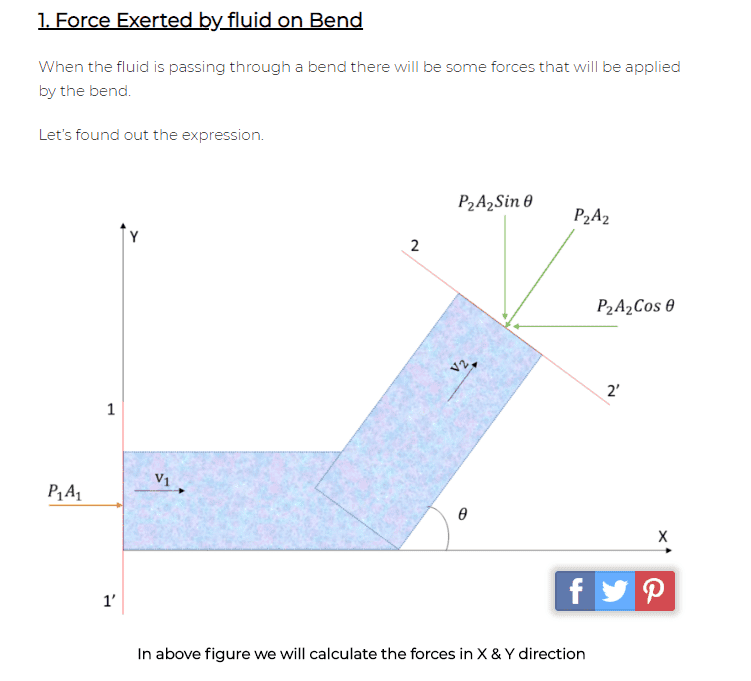# Understanding forces acting on a pipe bend when fluid is passing through

tomtomtom1
TL;DR Summary
I am trying to understand the forces that are acting on a pipe bend when a fluid is passing through it.
Hello all

I am trying to understand the forces that are acting on a pipe bend when a fluid is passing through it.

I have been given the following diagram:-The bit i cannot get my head around is why does P2A2 point the way it does i.e. down towards the left shouldn't is point the opposite direction i.e up right?

The reason why i say that the arrow direction is opposite is because the fluid is flowing in that direction?

Can someone explain?

Thank you.

Mentor
The force on A2 shown is the force acting on the fluid in the control volume exerted by the fluid downstream of the exit cross section.

•Lnewqban
Homework Helper
Gold Member
What force makes the fluid move in that direction, assuming the cross area remains the same?

Mentor
What force makes the fluid move in that direction, assuming the cross area remains the same?
The force of the pipe bend on the fluid and (of course) the forward pressure force at the entrance.